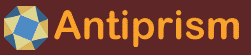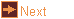# bravais - Bravais lattices

 Usage    |    Examples    |    Notes

## Usage

```

Usage: bravais [options] crystal_system [centering]

Generate Bravais lattices in OFF format. lattice may be specified by its index
number or (start of the) crystal_system name and centering

Crystal System   Centering   Vector Constraints  Angle Constraints
Triclinic        P           no constraints      any not of higher symmetries
Monoclinic       P,C         no constraints      alpha = gamma = 90 <> beta
Orthorhombic     P,C,F,I     a <> b <> c         alpha = beta = gamma = 90
Tetragonal       P,I         a = b <> c          alpha = beta = gamma = 90
Trigonal         P           a = b = c           alpha = beta = gamma <> 90
Hexagonal        P           a = b, c            alpha = beta = 90, gamma = 120
Cubic            P,F,I       a = b = c           alpha = beta = gamma = 90

Centering Types: P - Primitive (Simple) centering on corners (default: P)
C - Base (also A or B)  F - Face centering  I - Body centering

Synonyms: Rhombohedral = Trigonal  sc = Cubic P  fcc = Cubic F  bcc = Cubic I

Options
-h,--help this help message (run 'off_util -H help' for general help)
--version version information
-W        verbose output
-l <lim>  minimum distance for unique vertex locations as negative exponent
(default: 12 giving 1e-12)
-o <file> write output to file (default: write to standard output)

Lattice Options
-v <v,n>  vector lengths, non-zero, in form "a,b,c" (default: calculated)
optional fourth number, vectors taken to root n
-a <angs> angles in the form "alpha,beta,gamma". Ignored for Orthorhombic,
Tetragonal, and Cubic. For Hexagonal, any non-90 position may be
120. Otherwise, if not supplied then random angles are chosen.
Angles cannot be zero or 180. Angles may be negative values.
alpha + beta + gamma must be less than 360. Each angle must be
less than or equal to the sum of the other two angles
-g <grid> cell grid array. one or three positive integers separated by commas
a - automatic, of sufficient size for radius (-G required)
one integer, NxNxN grid. (default: calculated)
three integers, IxJxK grid. Combinations for grid center:
even,even,even - on cell corner    odd,odd,odd - in cell body
even,odd,even - on cell mid-edge   odd,even,odd - on face center
-G <type> automatic grid center type (type 8 invalid for cell centering = P):
p - corner, i - body, f - face, e - mid-edge, 8 - eighth cell
-I        inversion (centering type F or I)
-d <vrts> output dual of lattice based on primitive vectors
c - use primitive vectors base on centering type
four integers - primitive vectors are determined by four vertex
numbers given by non negative integers. The first vertex
number is the radial point and the next three vertices are the
primitive vectors
-s <s,n>  create struts. s is strut length taken to optional root n
use multiple -s parameters for multiple struts
-D <opt>  Voronoi (a.k.a Dirichlet) cells (Brillouin zones for duals)
c - cells only, i - cell(s) touching center only
-A        append the original lattice to the final product

Container Options
-c <type> container s - sphere (uses radius) (default: none)
-k <file> container, convex hull of off file or built in model (uses radius)
-r <c,n>  radius. c is radius taken to optional root n. n = 2 is sqrt
or  l - max insphere radius, s - min insphere radius (default)
or  k - take radius from container specified by -k
-p <xyz>  radius to lattice, three comma separated coordinates, 0 for origin
-q <xyz>  center offset, three comma separated coordinates, 0 for origin
-C <opt>  c - convex hull only, i - keep interior

Coloring Options (run 'off_util -H color' for help on color formats)
-V <col>  vertex color, (optional) transparency, (optional) elements
transparency: valid range from 0 (invisible) to 255 (opaque)
elements to color are l - lattice, c - convex hull, v - voronoi
h - hex relation (default elements: lcvh)
-E <col>  edge color (same format as for vertices)
-F <col>  face color (same format as for vertices) or
keyword: s,S color by symmetry using face normals
keyword: c,C color by symmetry using face normals (chiral)
lower case outputs map indexes. upper case outputs color values
-T <tran> face transparency for color by symmetry. valid range from 0 to 255

Scene Options
-R <opt>  hexagonal/cubic relation (Cubic P or Trigonal only)
o - hex overlay, f - hex fill, O - cube overlay, F - cube fill
-O        translate centroid of final product to origin
-Z <col>  add centroid vertex to final product in color col
-K        append cage of container of -k to final product

Listing Options
-B        display the list of Bravais lattices
-Q <vecs> center for radius calculations in -L (default: centroid)
c - original center, o - original center + offset in -q
-L <opt>  list unique radial distances of points (to standard output)
f - full report, v - values only
-S <opt>  list every possible strut value (to standard output)
f - full report, v - values only

```

## Examples

Make a cubic section of CCP
```bravais fcc | antiview -v b
```

Make a spherical section of BCC with struts from centre to vertex
```bravais bcc -c s -r 4 -s 3,2 | antiview
```

Make the Voronoi cells of a lattice
```bravais bcc -g 3 -D c | antiview
```

Add the Voronoi cells to a lattice
```bravais bcc -g 3 -D c -A | antiview -v 0.1
```

Make the dual of a lattice
```bravais bcc -d c | antiview -v b
```

Make a triclinic lattice, include the cell grid
```bravais triclinic -a 60,70,80 -v 1,1.5,2 -u | antiview
```

## Notes

bravais was written by Roger Kaufman.

The following extended help for the program may be displayed with bravais -H

```

Definition: (partly from http://en.wikipedia.org/wiki/Bravais_lattice)
In geometry and crystallography, a Bravais lattice, named after Auguste
Bravais, is an infinite set of points generated by a set of discrete
translation operations. A crystal is made up of one or more atoms (the basis)
which is repeated at each lattice point. The crystal then looks the same when
viewed from any of the lattice points. In all, there are 14 possible Bravais
lattices that fill three-dimensional space.

August Bravais (1811-1863), a French naval officer, adventurer, and physicist
taught a course in applied mathematics for astronomy in the faculty of sciences
in Lyon from 1840. He served as the Chair of Physics, Ecole Polytechnique
between 1845 and 1856. He is best remembered for pointing out in 1845, that
there are 14 unique Bravais lattices in three dimensional crystalline systems,
adjusting the previously result (15 lattices) by Moritz Ludwig Frankenheim
obtained three years before.

A German Crystallographer, Frankenheim (1801-1869) is noted as the first to
enumerate the 32 crystal classes. And he also solved the symmetry systems of
the 7 crystal systems but this work went completely unnoticed at the time.
There is a bit of mystery surrounding what Frankenheim had as the 15th lattice.
Even today, in some texts the Hexagonal lattice with two interior points is
shown in the Trigonal class. But these two lattices use the same set of points
and it is thought that it was this duplication that was eliminated by Bravais.
However, in Bravais' paper, there is no mention of Frankenheim or the
enumeration of lattices he presented.

In this program, the Hexagonal cells and Trigonal cells can be seen together
by using the -R parameter.

Note that End Centered Cubic (would be Cubic C) does not exist but can be
produced by Tetragonal P that has cells of dimensions a,b,c = 1,1,sqrt(2)

Face Centered Cubic (Cubic F or FCC) is duplicated in Body Centered Tetragonal
(Tetragonal I) of dimensions a,b,c = 1,1,sqrt(2). However, the FCC embodied
would be of higher symmetry than the Tetragonal crystal system is allowed.

Similarly, Trigonal at 90 degrees (improper) is SC. Trigonal at 60 degrees is
FCC and Trigonal at acos(-1/3) or 109.47122063449... degrees is BCC.

Also there is no provision for Face Centered Tetragonal (would be Tetragonal F)
or Base Centered Tetragonal (would be Tetragonal C). These would be embodied in
Body Centered Tetragonal (Tetragonal I) and Simple Tetragonal (Tetragonal P)
respectively. This is true at any proportion other than a,b,c = 1,1,sqrt(2)

In Hexagonal, Orthorhombic C can be seen to occur. When Hexagonal of a=b=c is
produced, then Base Centered Tetragonal (would be Tetragonal C) occurs.

Hexagonal is sensitive to which unequal vector corresponds to the non-90 degree
angle. These must be in the same position or a Monoclinic lattice is produced.

Bravais lattices will fall into the following symmetries

Crystal System   Possible Symmetries (32 possible - note no 5 fold symmetries)
Triclinic        C1 Ci
Monoclinic       C2 Cs C2h
Orthorhombic     D2 C2v D2h
Tetragonal       C4 S4 C4h D4 C4v D2d D4h
Trigonal         C3 S6 D3 C3v D3d
Hexagonal        C6 C3h C6h D6 C6v D3h D6h
Cubic            T Th O Td Oh
Of the symbols used for cell centering:
P - stands for Primitive. It is a cube depicted by a vertex at eight corners
C - stands for having a point filled in on the "C" side of the primitive cell
this is described in some texts as Base Centering. C is most commonly used
A or B means use the "A" or "B" sides instead. Just a rotation of C
F - stands for Face Centering and fills all three, "A", "B" and "C" sides
I - (from German: innenzentriert, meaning Body Centered) is the primitive cell
with one point filled in the center of the cell

The term Isometric is sometimes used for Cubic. Also allowable in this program.

Monoclinic is defined in this program with angles alpha = gamma = 90 <> beta
as is found in the first volume of International Tables for Crystallography.

Vectors a, b and c correspond to axes x, y and z (before transformations).
```